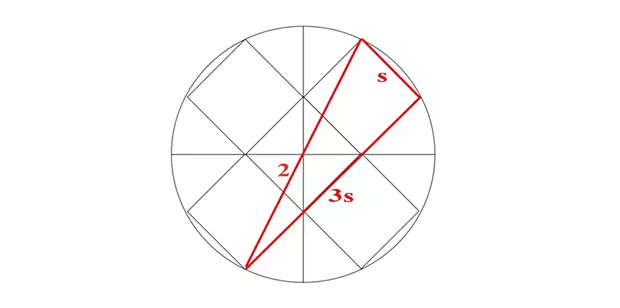comment

Here are the solutions to last week's challenge. If you haven't tried it yet, don't peek — go to www.northcoastjournal.com to find the questions online.

The Mistake

It's the extra "the."

Pedal to the Metal

Infinitely fast. The car needs two minutes to drive two miles at 60 mph. But those two minutes have already been used up in the first mile driving at 30 mph. So, it's impossible.

Four Fours

All have multiple answers but I believe these are the shortest.

0 = 44 − 44 1 = 44 / 44 2 = 4/4 + 4/4 3 = (4 + 4 + 4) / 4 4 = 4 × (4 − 4) + 4 5 = (4 × 4 + 4) / 4 6 = 4 × .4 + 4.4 7 = 44 / 4 − 4 8 = 4 + 4.4 − .4 9 = 4/4 + 4 + 4 10 = 44 / 4.4 11 = 4/.4 + 4/4 12 = (44 + 4) / 4 13 = 4! − 44/4

Special Words

They're pronounced differently when the first letter is capitalized.• Submitted
Arc-square

0.4. Let s be the length of a side of the square. Fill in the remaining three quadrants as shown and connect the top left corner of the original square with the bottom right of the opposite square, which (by symmetry) passes through the center of this circle, i.e. it's a diameter, length 2. By Pythagoras, s^2 + (3s)^2 = 2^2, that is, 10s^2 = 4, so s^2, the area of the blue square, is 4/10.

Matches

Square root of 1; and using Roman numerals for 11.

Barry Evans (he/him, barryevans9@yahoo.com) welcomes any puzzle ideas for future use in Field Notes.